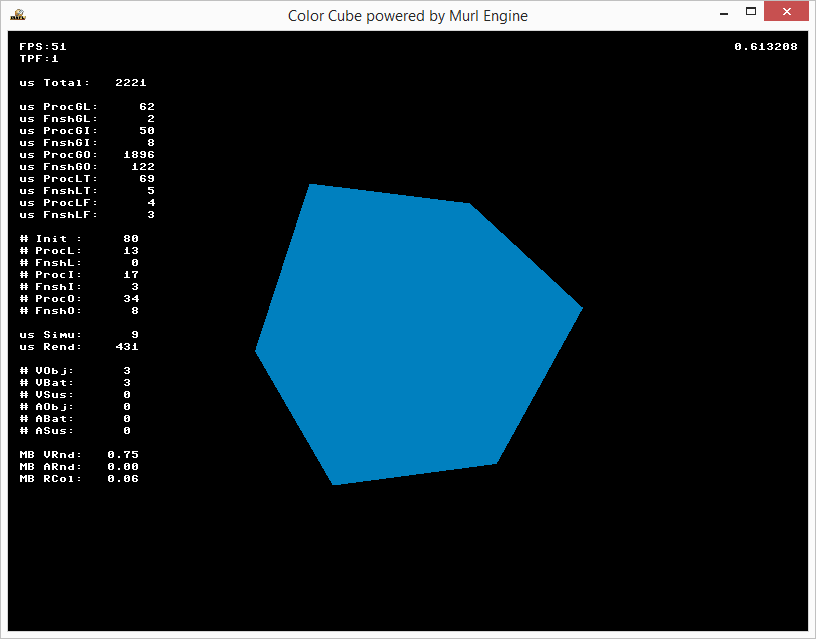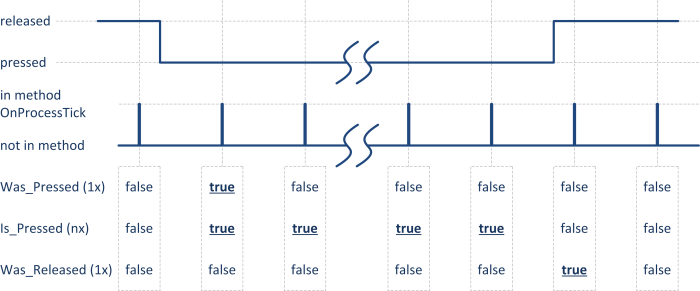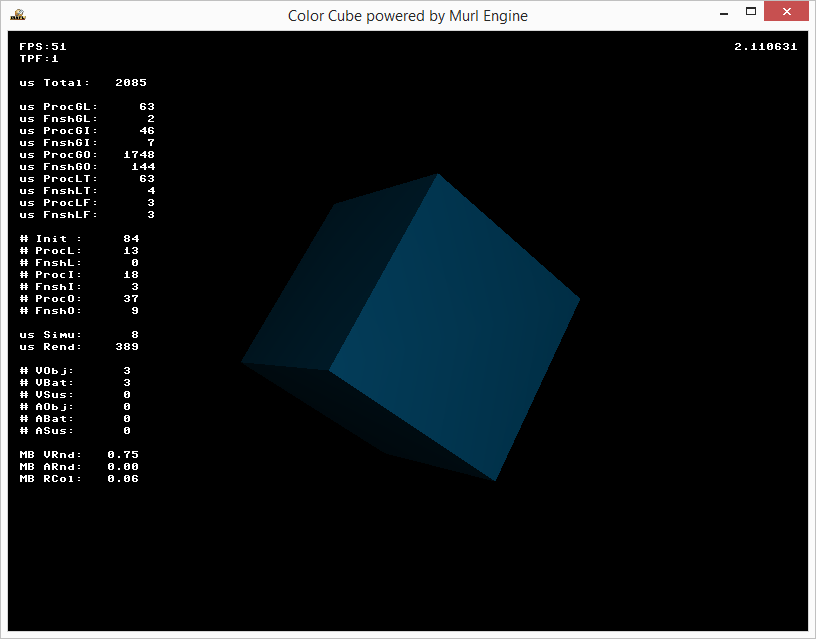Tutorial #02: Color Cube

In the next tutorial, we add a colored material and a light source to make our cube look a bit more interestingly and extend our logic processor to react on user key input. We will take the previous tutorial’s code as the basis for our next steps.

Find the project files in the folder `tutorials/chapter01/02_color_cube/project`.

# Version 1: Structuring Graph Description Files

For reasons of clarity, we start by moving the definition of our cube’s material to a new file named `graph_materials.xml`. Splitting logical groups to multiple files is useful especially in larger projects and allows the easy reuse of existing components.

## Namespaces

To avoid naming conflicts, it is possible to use `Graph::Namespace` nodes in our scene graph, which represent a way to hierarchically organize our graph nodes.

Similar to file paths in a computer file system, a named reference into the scene graph can be represented by a (nested) representation of namespace IDs, followed by the actual node ID. The `/` character serves as a separator, much like in an UNIX-like file system. A preceding `/` character denotes the root namespace analogous to an absolute file path. Without a preceding `/` , the referenced element can be specified relative to the current namespace. Moving up one level can be done using the character sequence `../` .

```<MaterialState materialId="/material/mat_white">
<MaterialState materialId="../material/mat_white">
<MaterialState materialId="/material/uncolored/mat_white">
```

The above notation is used for referencing nodes within the loaded scene graph. Referencing individual resources from a loaded package works in a similar way. However, in this case it is necessary to specify the actual package by preceding its ID to the given resource ID. Here, the `:` character serves as separator:

```<Instance graphResourceId="package_main:graph_mat"/>
```

In our example, the material’s path changes from `"mat_white"` to `"/material/mat_white"` when located in the `"material"` namespace, and `"myCube01"` changes to `"/myGraph/myCube01"` .

We also specify the optional attribute `activeAndVisible="no"` at the namespace node to deactivate and hide all nodes in its sub-tree. We will later see why.

```<?xml version="1.0"?>
<Graph>
<Namespace id="material" activeAndVisible="no">
<FixedProgram
id="prg_white"
/>
<Material
id="mat_white"
programId="prg_white"
/>
</Namespace>
</Graph>
```

We also change the file `graph_main.xml` to:

```<?xml version="1.0" ?>
<Graph>
<Instance graphResourceId="package_main:graph_mat"/>
<Namespace id="myGraph">
<View id="view"/>
<Camera id="camera"
viewId="view"
fieldOfViewX="400"
nearPlane="400" farPlane="2500"
clearColorBuffer="1"
>
<CameraTransform
cameraId="camera"
posX="0" posY="0" posZ="800"
/>
<MaterialState
materialId="/material/mat_white"
slot="0"
/>
<CubeGeometry id="myCube01"
scaleFactor="200"
posX="0" posY="0" posZ="0"
/>
</Camera>
</Namespace>
</Graph>
```

Both resource files must be defined in the file `package.xml` in order to include them in the package:

```<?xml version="1.0" ?>

<Package id="package_main">

<!-- Graph resources -->
<Resource id="graph_main" fileName="graph_main.xml"/>
<Resource id="graph_mat" fileName="graph_materials.xml"/>

<!-- Graph instances -->
<Instance graphResourceId="graph_main"/>

</Package>
```

We can use the `Graph::IRoot::PrintTree()` method to print the whole sub-graph starting at a given node to the console. Comparing this output to the output of the previous example shows the actually created `Namespace` node: Additionally, it shows that the whole graph is embedded into a root namespace node.

```<Namespace flags=e000011e vnode="2">
<Container flags=e000011d vnode="1">
<Instance flags=8000011e vnode="5">
<Container flags=8000011e vnode="4">
<Namespace id="material" flags=80000186 vnode="3">
<FixedProgram id="prg_white" flags=0000001e vnode="1"/>
<Material id="mat_white" flags=8000001e vnode="1"/>
<Namespace/> <!-- id="material" -->
<Container/>
<Instance/>
<Namespace id="myGraph" flags=e000011e vnode="6">
<View id="view" flags=e000001e vnode="1"/>
<Camera id="camera" flags=e000011e vnode="4">
<CameraTransform flags=4000001e vnode="1"/>
<MaterialState flags=8000001e vnode="1"/>
<CubeGeometry id="myCube01" flags=8000001e vnode="1"/>
<Camera/> <!-- id="camera" -->
<Namespace/> <!-- id="myGraph" -->
<Container/>
<Namespace/>
```
```<Namespace flags=e000011e vnode="2">
<Container flags=e000011d vnode="1">
<View id="view" flags=e000001e vnode="1"/>
<Camera id="camera" flags=e000011e vnode="6">
<CameraTransform flags=4000001e vnode="1"/>
<FixedProgram id="prg_white" flags=0000001e vnode="1"/>
<Material id="mat_white" flags=8000001e vnode="1"/>
<MaterialState flags=8000001e vnode="1"/>
<CubeGeometry id="myCube01" flags=8000001e vnode="1"/>
<Camera/> <!-- id="camera" -->
<Container/>
<Namespace/>
```

When the `root->PrintTree()` method is called from `OnInit()`, it is possible that there are still some nodes remaining in the graph that actually belong to the `startup` package, even if `UnloadPackage("startup")` has already been called. The `UnloadPackage()` method simply puts the respective nodes in an inactive state and delegates the actual unloading process to a different thread. To receive a notification, when the package has been unloaded, the callback method `OnPackageWasUnloaded()` has to be overridden. There exist a total number of four callback methods for the purpose of signalling package loading states:

# Version 2: Defining a Colored Material

In the next step, we define a new `FixedProgram` using the attribute value `coloringEnabled="yes"`. In addition, we specify a material using default values, and a new `FixedParameters` node together with its `diffuseColor` attribute.

Depending on the format and suffix(es) given for that attribute, a color value can be specified in a number of different ways:

Suffix Range Type Format
f 0.0 – 1.0 floating point e.g. 0f, 0.50f, 0.75f, 1f RGBA / RGB
i 0 – 255 integer e.g. 0i, 128i, 191i, 255i RGBA / RGB
h 00 – FF 8 bit hex int e.g. 0h, 80h, BFh, FFh RGBA / RGB
h 0 – FFFFFFFF 32 bit hex int e.g. FF0080BFh ARGB / RGB
```        <FixedProgram
id="prg_color"
coloringEnabled="yes"
/>
<Material
id="mat_color"
programId="prg_color"
/>
<FixedParameters
id="par_cube_color"
diffuseColor="0f, 0.50f, 0.75f, 1f"
/>
```

In the file `graph_main.xml` we now have to activate both our new material and parameter nodes for rendering. We could also safely omit the given attribute definition `slot="0"` as the default value for the slot attribute is 0 in any case.

```            <CameraTransform
cameraId="camera"
posX="0" posY="0" posZ="800"
/>
<MaterialState
materialId="/material/mat_color"
slot="0"
/>
parametersId="/material/par_cube_color"
slot="0"
/>
<CubeGeometry id="myCube01"
scaleFactor="200"
posX="0" posY="0" posZ="0"
/>
```

As a result, our cube gets rendered using the selected color:Color Cube V2 output window showing the spinning color cube

# Version 3: Defining a Light Source

To enable simple lighting for our cube, we have to perform two additional steps:

Material lighting calculations can be simply enabled by using the `lightingEnabled="yes"` attribute:

```        <FixedProgram
id="prg_color"
coloringEnabled="yes"
lightingEnabled="yes"
/>
```

So far, we always added our cube as a child node of our camera to assign it to the camera for rendering. A generally more flexible way to perform this task is to use a `CameraState` node, which allows separating the definitions of camera and geometry. Analogous to material and parameters states, a `CameraState` node activates a camera for all subsequent rendering until a different `CameraState` node is encountered.

```<?xml version="1.0" ?>

<Graph>
<Instance graphResourceId="package_main:graph_mat"/>

<Namespace id="myGraph">
<View id="view"/>
<Camera
id="camera"
viewId="view"
fieldOfViewX="400"
nearPlane="400" farPlane="2500"
clearColorBuffer="1"
/>
<CameraTransform
cameraId="camera"
posX="0" posY="0" posZ="800"
/>
<CameraState
cameraId="camera"
/>
```

In order to define a single light source object, we need another three graph nodes:

The `Light` node is used to create an actual light source. The `LightTransform` node is used to position the light in our virtual world; in our case, we position it at the same position as out camera. The `LightState` node activates our light for all subsequent rendering operations:

```        <Light
id="light"
/>
<LightTransform
lightId="light"
posX="0" posY="0" posZ="800"
/>
<LightState
lightId="light"
unit="0"
/>
```

Now we can render our cube using a suitable material:

```        <MaterialState materialId="/material/mat_color"/>
<CubeGeometry
id="myCube01"
scaleFactor="200"
posX="0" posY="0" posZ="0"
/>
</Namespace>
</Graph>
```

The result is a rotating cube with simple lighting enabled:Color Cube V3 output window showing the spinning color cube with simple lighting

# Version 4: Device Handler

To conclude this example, we want to be able to close our application, when the user presses the ESC key on a physical keyboard or the BACK button on an Android device, and furthermore, we also want to improve the color parameters for lighting.

To react on different user input, the Murl engine provides a device handler object (`Logic::IDeviceHandler`). We can obtain a pointer to this object using the `Logic::IState::GetDeviceHandler()` method. To check for physical keyboard input, we can use the device handler's `WasRawKeyPressed()` method; on Android buttons we can use `WasRawButtonPressed()`.

In addition to `WasRawKeyPressed()`, physical keyboard input is also reflected by the `IsRawKeyPressed()` and `WasRawKeyReleased()` methods. During a regular keystroke, these methods return true in the following sequence:

This scheme (was pressed, is pressed, was released) can be found throughout the Murl engine, e.g. for `Input::IRawKeyboardDevice`, `Input::IRawButtonDevice`, `Input::IJoystickDevice`, `Input::IMouseButtons` etc.Pressed/released input states
```void App::ColorCubeLogic::OnProcessTick(const Logic::IState* state)
{
Double angle = state->GetCurrentTickTime();
angle = Math::Fmod(angle, Math::TWO_PI);
mCubeTransform->SetRotation(angle, angle, 0);
state->SetUserDebugMessage(Util::DoubleToString(angle));

Logic::IDeviceHandler* deviceHandler = state->GetDeviceHandler();
if (deviceHandler->WasRawKeyPressed(RAWKEY_ESCAPE) ||
deviceHandler->WasRawButtonPressed(RAWBUTTON_BACK))
{
deviceHandler->TerminateApp();
}
}
```

Finally, we can change the result of our cube’s lighting calculations by modifying the color values for both light source and parameter node for one or more of the individual components of the lighting equation:

```        <Light
id="light"
diffuseColor="0.5f,0.5f,0.5f,1f"
/>
```
```        <FixedProgram
id="prg_color"
coloringEnabled="yes"
lightingEnabled="yes"
/>
<Material
id="mat_color"
programId="prg_color"
/>
<FixedParameters
id="par_cube_color"
diffuseColor="0f, 0.50f, 0.75f, 1f"
specularColor="1f, 1f, 1f, 1f"
ambientColor="0f, 0f, 0f, 1f"
shininess="32"
/>
```Color Cube V4 output window showing the spinning color cube with lighting

## Exercises

• Try different color parameter values.
• What needs to be done to rotate the cube manually around the X, Y and Z axes using the keyboard keys 1, 2 and 3 respectively instead of automatic rotation?